# Chapter 6 Chemical Reactions Section 2 Classifying Chemical

• Slides: 22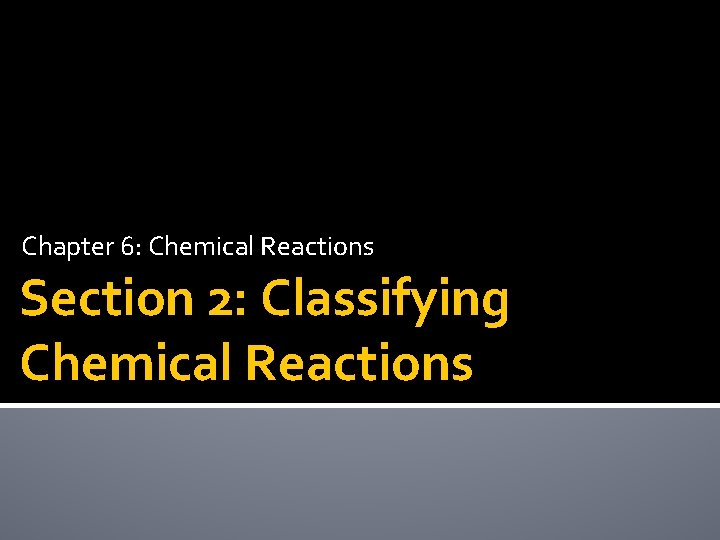Chapter 6: Chemical Reactions Section 2: Classifying Chemical ReactionsLearning Goals �Classify chemical reactions.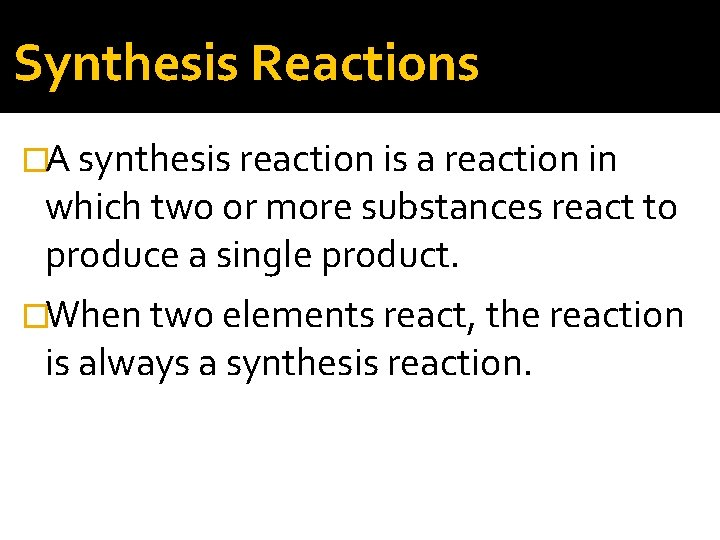Synthesis Reactions �A synthesis reaction is a reaction in which two or more substances react to produce a single product. �When two elements react, the reaction is always a synthesis reaction.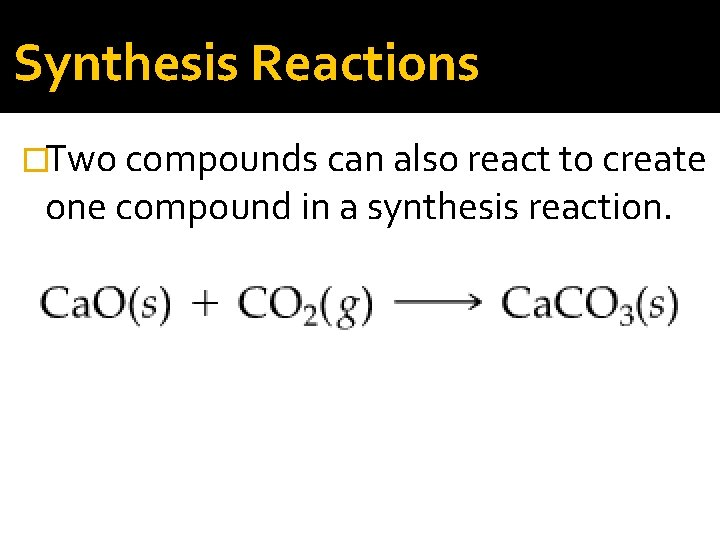Synthesis Reactions �Two compounds can also react to create one compound in a synthesis reaction.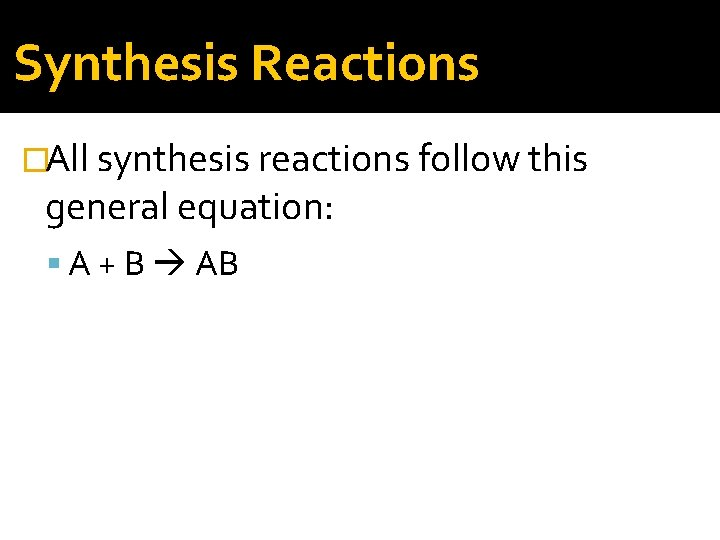Synthesis Reactions �All synthesis reactions follow this general equation: A + B ABDecomposition Reactions �A decomposition reaction is one in which a single compound breaks down into two or more elements or new compounds.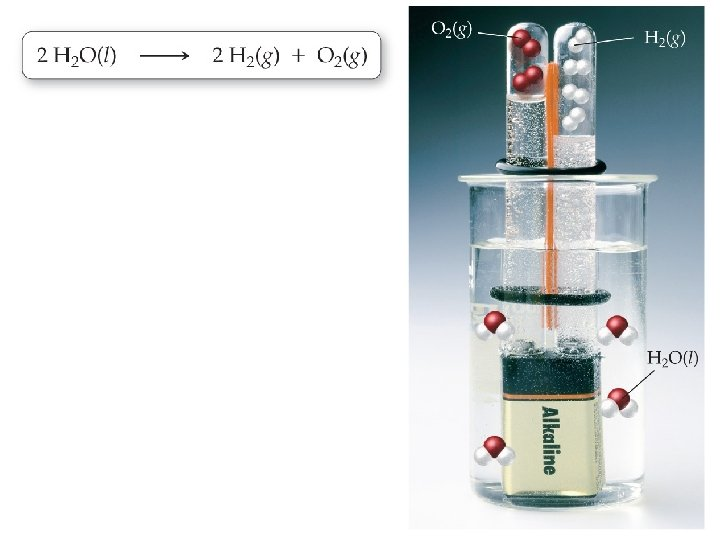Decomposition Reactions �Decomposition reactions often require an energy source, such as heat, light, or electricity, to occur. �Decomposition reactions follow this general equation: AB A + B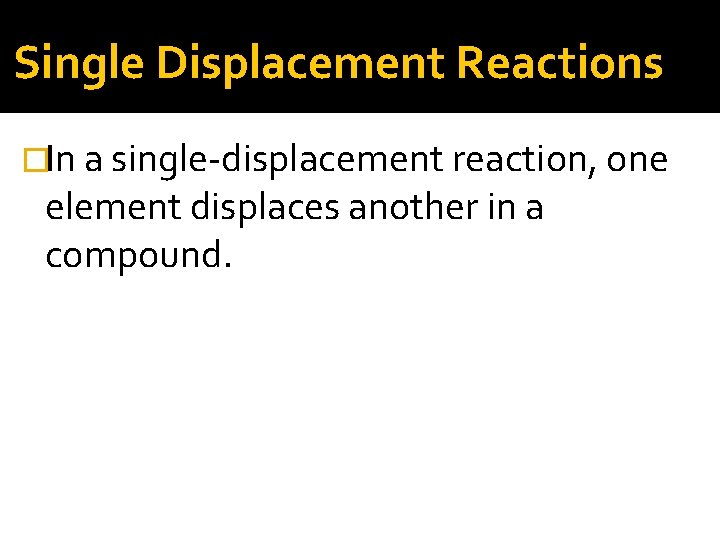Single Displacement Reactions �In a single-displacement reaction, one element displaces another in a compound.Single Displacement Reactions �Usually a metal will replace another metal, or a halogen will replace another halogen in single displacement reactions �Single displacement reactions follow this general equation: A + BC AC + B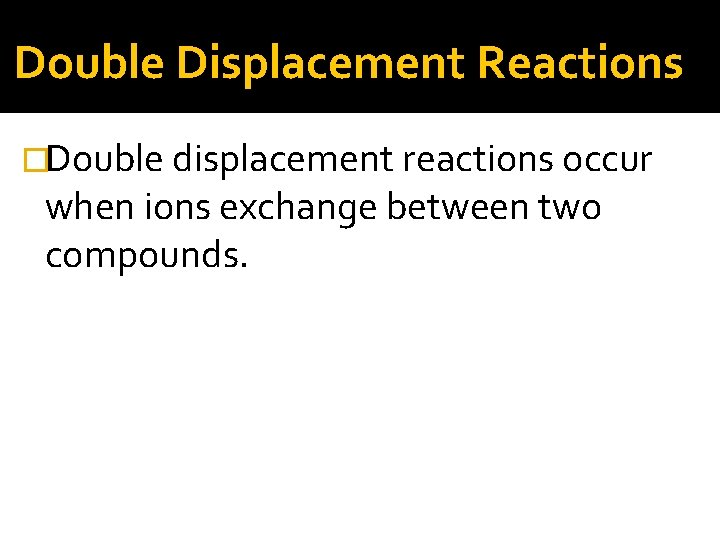Double Displacement Reactions �Double displacement reactions occur when ions exchange between two compounds.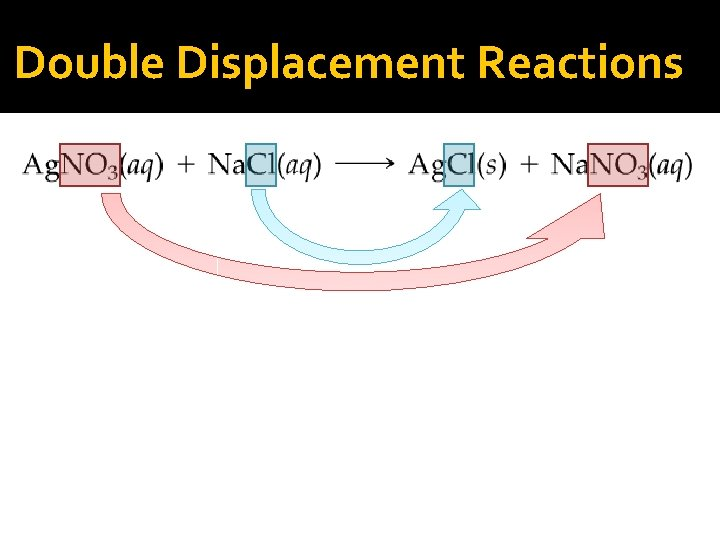Double Displacement Reactions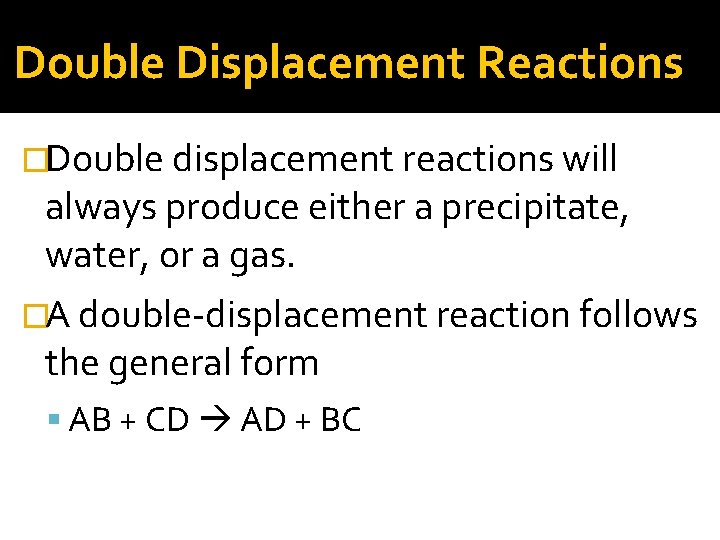Double Displacement Reactions �Double displacement reactions will always produce either a precipitate, water, or a gas. �A double-displacement reaction follows the general form AB + CD AD + BC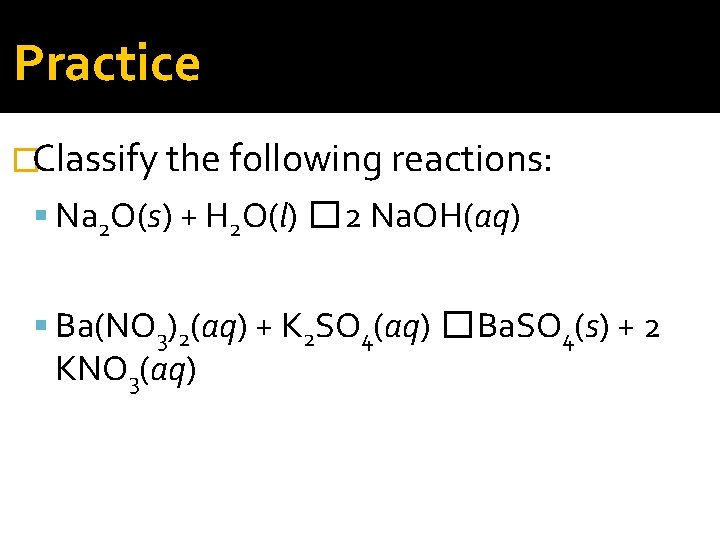Practice �Classify the following reactions: Na 2 O(s) + H 2 O(l) � 2 Na. OH(aq) Ba(NO 3)2(aq) + K 2 SO 4(aq) � Ba. SO 4(s) + 2 KNO 3(aq)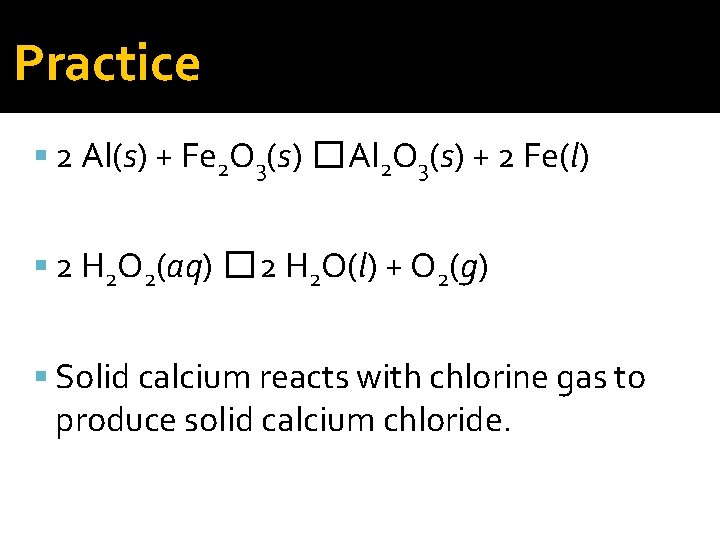Practice 2 Al(s) + Fe 2 O 3(s) � Al 2 O 3(s) + 2 Fe(l) 2 H 2 O 2(aq) � 2 H 2 O(l) + O 2(g) Solid calcium reacts with chlorine gas to produce solid calcium chloride.Practice �Write a balanced chemical equation for each synthesis reaction: Ag(s) + Br 2(g) Mg(s) + Cl 2(g)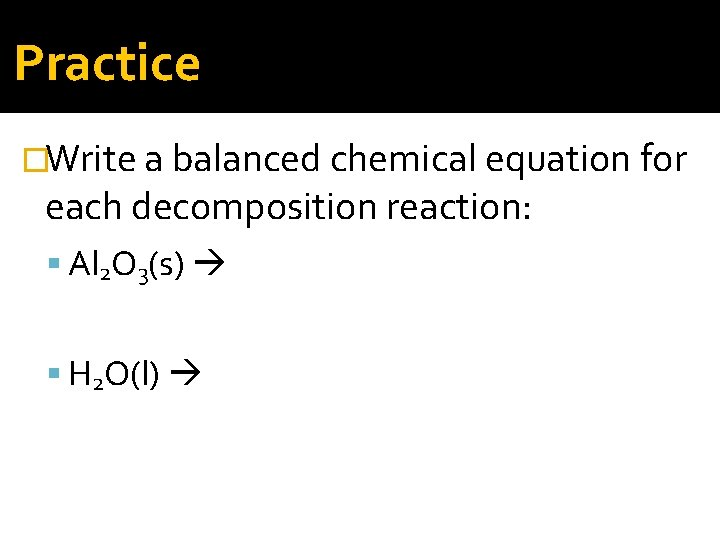Practice �Write a balanced chemical equation for each decomposition reaction: Al 2 O 3(s) H 2 O(l)